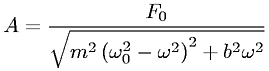Equations > Physics > Oscillations and Waves > Amplitude of a driven oscillation

### Amplitude of a driven oscillationLatex Code:

MathML Code:

 $A=\frac{F}_{0}\sqrt{{m}^{2}{\left({\omega }_{0}^{2}-{\omega }^{2}\right)}^{2}+{b}^{2}{\omega }^{2}}$

MathType 5.0: